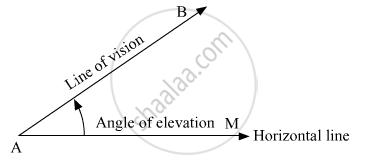Share

# Choose the Correct Alternative Answer for the Following Question. When We See at a Higher Level, from the Horizontal Line, Angle Formed is ........ - Geometry

ConceptApplication of Trigonometry

#### Question

Choose the correct alternative answer for the following question.

When we see at a higher level, from the horizontal line, angle formed is ........

• angle of elevation.

• angle of depression.

• 0

• straight angle.

#### Solution

When we see at a higher level, from the horizontal line, angle formed is angle of elevation.Hence, the correct answer is angle of elevation.

Is there an error in this question or solution?

#### APPEARS IN

Solution Choose the Correct Alternative Answer for the Following Question. When We See at a Higher Level, from the Horizontal Line, Angle Formed is ........ Concept: Application of Trigonometry.
S